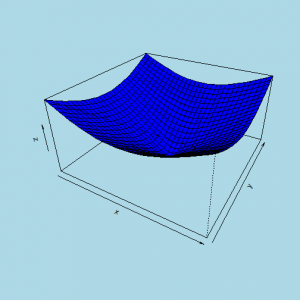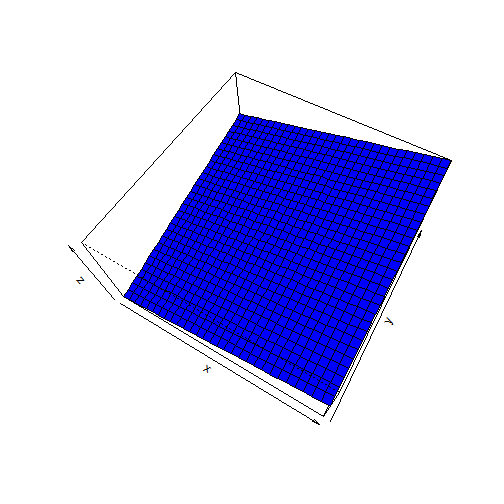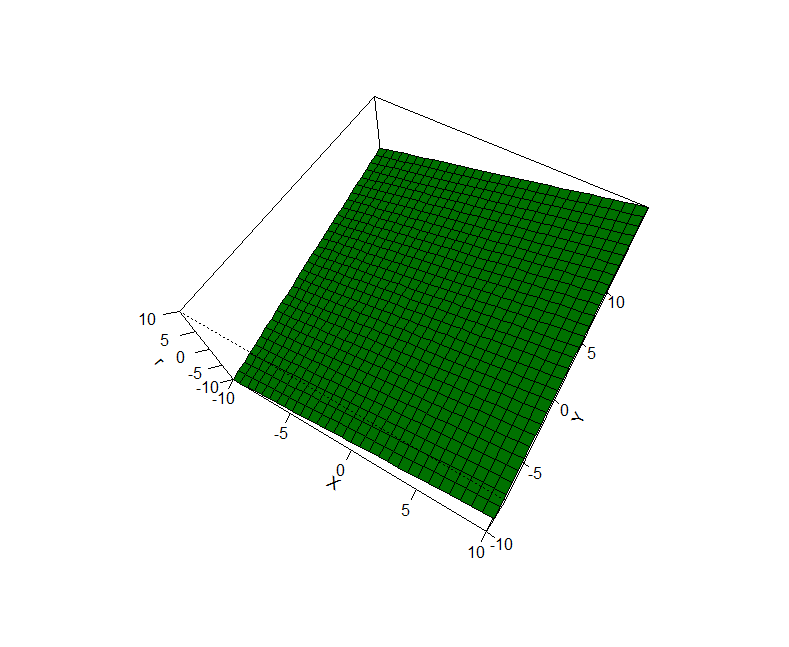Want to share your content on R-bloggers? click here if you have a blog, or here if you don't.In this set of exercises we will introduce the concept of 3D plotting. Specifically, we will use these commands:`image()`, `contour()` and `persp()`.

For these exercises, you need to have a basic understanding of R objects and functions, in particular some knowledge about ` matrix `.

This set is the fourth set of exercises is a series on data visualization. Click here for the other sets in the series. Answers to the exercises are available here.

If you obtained a different (correct) answer than those listed on the solutions page, please feel free to post your answer as a comment on that page.

Exercise 1
Construct a matrix of 10 rows by 10 columns filled with random numbers between 0 and 1, uniformly distributed. Plot it using the ` image() ` function.

Exercise 2
Costumize the plot by changing from colours to grayscale and equally space the squares.

Exercise 3
Construct a matrix of 5 rows by 5 columns with random numbers as in exercise 1, and create a contour plot.

Exercise 4
To create a 3d plot with the `persp` function, we need 3 variables (x,y,z) where z is a matrix that contains values to be plotted. Try to replicate this plot (hint: the relation between x,y and z is defined by: z <- (x+y)/2:Exercise 5
Now let’s customize our 3d plot, modifying some details such as background colour and values colours. Then change the way to define x and y variables. The final result it should be like this:Exercise 6
Let’s take our last 3d plot and add some details as labels and plot orientation (hint: phi value). Final result should be like this:Exercise 7
For the final exercise we have to reproduce the 3d plot below using what we have learned in the previous exercises: